### Objective Questions on Basics of Electronics

Page 1

In this section we will cover objective questions or MCQs on Basics of Electronics. Just start from this section and gradually you will move forward with better confidence to achieve desired results in job oriented exams.

• Diameter of an atom is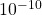• The electrons in the last orbit of an atom are called valence electrons

• The number of compounds available in nature is unlimited

• If the of no. of valence electrons of an atom is less than 4, the substance is usually a conductor

• If the of no. of valence electrons of an atom is more than 4, the substance is usually an insulator

• When number of valence electrons of an atom is 4, the substance is usually semiconductor.

• One coulomb of charge is equal to the charge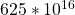electrons

• I cm3 of copper at room temperature has about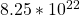free electrons

• The electric current is due to the flow of both positive and negative charges

• E.M.F in a circuit maintains potential difference.

• The electric current in a metallic conductor is due to the flow of electrons only

• Potential difference has the unit of energy

• The electric current in a liquid is due to the flow of negative and positive ions both

• Electric current is a scalar quantity

• A billion electrons pass through a cross-section of a conductor in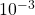s. The current is 1.6x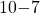A.

• The electric current in a discharge tube containing a gas is due to electrons and positive ions both

• How long does it take 50 µC of charge to pass a point in a circuit if the current flow is 15 mA? 3.33 x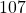s

• The specific resistance of a wire depends upon its material

• The quantity of charge that will be transferred by a current flow of 10 A over 1 hour period is 3.6 x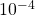A.

• The resistance of a straight conductor is independent of shape of cross-section

• A length of wire has a resistance of 6 ohms. The resistance of a wire of the same maternal three
times as long and twice the cross-sectional area will be 9 ohms.

• The drift velocity of electrons is of the order of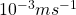• Conductivity is the reciprocal of resistivity

• The specific resistance of a metallic conductor increases with rise in temperature

• The potential difference of an energy source that provides 50 mJ of energy for every micro coulomb
of charge that flows is 5O0kV

• Insulators have negative temperature co-efficient of resistance

• Eureka has almost zero temperature co-efficient of resistance.

• What quantity of charge must be delivered by a battery with a potential difference of 100 V to do
500 J of work? 5C

• A piece of aluminum wire is stretched to reduce its diameter to half of its original value. Its
resistance will become sixteen times

• Semiconductors have negative temperature coefficient of resistance

• The thermal speed of electrons is of the order of 10° ms”

• Constantan wire is used for making standard resistance because it has negligibly small temperature co-efficient of resistance